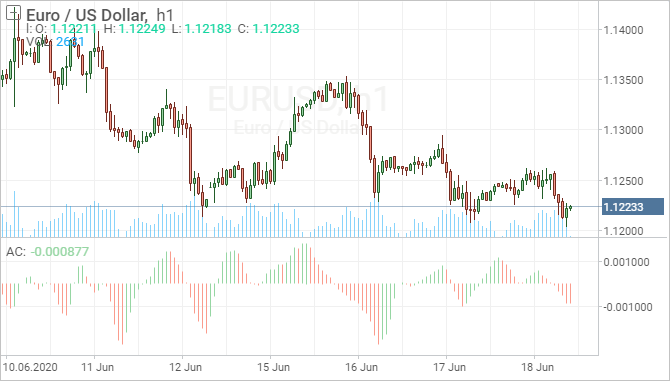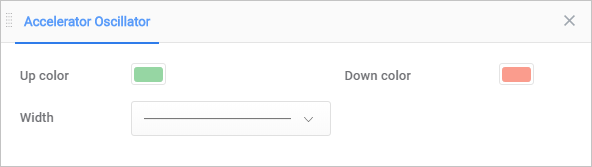Overview

Bill Williams, a well-known technical analyst, developed 5 measurements for technical analysis, the third of which is used to determine accelerator or decelerator of the driving force. Awesome Oscillator enable traders to determine the momentum, but for interpreting its force more accurately, Accelerator Oscillator is required accordingly.

Acceleration/Deceleration Technical Indicator (AC) measures acceleration and deceleration of the current driving force, gauging an acceleration change in momentum. The direction of this indicator will always change before the price, meaning that looking at the indicator’s momentum instead of just the price gives a timing advantage. The Acceleration Indicator is seeking to go one step further and detect early changes in momentum – that is, when momentum is accelerating or decelerating.Description

Acceleration/Deceleration oscillator formula is based on values of another Williams indicator – Awesome Oscillator and shall be calculated as the difference between Awesome Oscillator and the 5-period moving average of Awesome Oscillator.

AC = AO — SMA (AO, 5)

At that

AO = SMA (MEDIAN PRICE, 5) — SMA (MEDIAN PRICE, 34),

MEDIAN PRICE = (HIGH + LOW) / 2

Where:

MEDIAN PRICE — median price;

HIGH — maximum bar price;

LOW — minimum bar price;

SMA — simple moving average;

AO — Awesome Oscillator indicator.

It should be noted that Bill Williams himself suggested using periods 5 and 34, but their practical application revealed a large number of false signals with such parameters, that is why each trader determines his parameters as he/she familiarizes with the oscillator.

The nought line is basically the spot where the driving force is at balance with the acceleration. It’s meaning that when the indicator is above the zero line, it is easier for acceleration to continue to increase. Conversely, when the indicator is below zero, it is easier for deceleration to increase. However, unlike the Awesome Oscillator, crossing the zero line is not a trading signal for investors in itself, but it gives a change in the pattern that will bolster optimism on placing a trade.

Settings in the chart